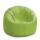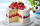# Fraction calculator

The calculator performs basic and advanced operations with fractions, expressions with fractions combined with integers, decimals, and mixed numbers. It also shows detailed step-by-step information about the fraction calculation procedure. Solve problems with two, three, or more fractions and numbers in one expression.

## Result:

### 6/12 + 4/6 = 7/6 = 1 1/6 ≅ 1.1666667

Spelled result in words is seven sixths (or one and one sixth).

### How do you solve fractions step by step?

1. Add: 6/12 + 4/6 = 6/12 + 4 · 2/6 · 2 = 6/12 + 8/12 = 6 + 8/12 = 14/12 = 2 · 7/2 · 6 = 7/6
For adding, subtracting, and comparing fractions, it is suitable to adjust both fractions to a common (equal, identical) denominator. The common denominator you can calculate as the least common multiple of both denominators - LCM(12, 6) = 12. In practice, it is enough to find the common denominator (not necessarily the lowest) by multiplying the denominators: 12 × 6 = 72. In the next intermediate step, , cancel by a common factor of 2 gives 7/6.
In words - six twelfths plus four sixths = seven sixths.

#### Rules for expressions with fractions:

Fractions - use the slash “/” between the numerator and denominator, i.e., for five-hundredths, enter 5/100. If you are using mixed numbers, be sure to leave a single space between the whole and fraction part.
The slash separates the numerator (number above a fraction line) and denominator (number below).

Mixed numerals (mixed fractions or mixed numbers) write as non-zero integer separated by one space and fraction i.e., 1 2/3 (having the same sign). An example of a negative mixed fraction: -5 1/2.
Because slash is both signs for fraction line and division, we recommended use colon (:) as the operator of division fractions i.e., 1/2 : 3.

Decimals (decimal numbers) enter with a decimal point . and they are automatically converted to fractions - i.e. 1.45.

The colon : and slash / is the symbol of division. Can be used to divide mixed numbers 1 2/3 : 4 3/8 or can be used for write complex fractions i.e. 1/2 : 1/3.
An asterisk * or × is the symbol for multiplication.
Plus + is addition, minus sign - is subtraction and ()[] is mathematical parentheses.
The exponentiation/power symbol is ^ - for example: (7/8-4/5)^2 = (7/8-4/5)2

#### Examples:

adding fractions: 2/4 + 3/4
subtracting fractions: 2/3 - 1/2
multiplying fractions: 7/8 * 3/9
dividing Fractions: 1/2 : 3/4
exponentiation of fraction: 3/5^3
fractional exponents: 16 ^ 1/2
adding fractions and mixed numbers: 8/5 + 6 2/7
dividing integer and fraction: 5 ÷ 1/2
complex fractions: 5/8 : 2 2/3
decimal to fraction: 0.625
Fraction to Decimal: 1/4
Fraction to Percent: 1/8 %
comparing fractions: 1/4 2/3
multiplying a fraction by a whole number: 6 * 3/4
square root of a fraction: sqrt(1/16)
reducing or simplifying the fraction (simplification) - dividing the numerator and denominator of a fraction by the same non-zero number - equivalent fraction: 4/22
expression with brackets: 1/3 * (1/2 - 3 3/8)
compound fraction: 3/4 of 5/7
fractions multiple: 2/3 of 3/5
divide to find the quotient: 3/5 ÷ 2/3

The calculator follows well-known rules for order of operations. The most common mnemonics for remembering this order of operations are:
PEMDAS - Parentheses, Exponents, Multiplication, Division, Addition, Subtraction.
BEDMAS - Brackets, Exponents, Division, Multiplication, Addition, Subtraction
BODMAS - Brackets, Of or Order, Division, Multiplication, Addition, Subtraction.
GEMDAS - Grouping Symbols - brackets (){}, Exponents, Multiplication, Division, Addition, Subtraction.
Be careful, always do multiplication and division before addition and subtraction. Some operators (+ and -) and (* and /) has the same priority and then must evaluate from left to right.

## Fractions in word problems:

• Roses and tulipsAt the florist are 50 tulips and 5 times fewer roses. How many flowers are in the flower shop?
• Integer add to fraction7 is added to the sum of 4/5 and 6/7
• Adding mixed numbersAdd this two mixed numbers: 1 5/6 + 2 2/11=
• Circular gardenAlice creates a circular vegetable garden. Tomatoes are planted in 1/3 of the circular garden, carrots are planted in 2/5 of the circular garden, and green peppers are planted in 1/10 of the circular garden. What fraction represents the remaining unplante
• A dumpA dump truck bought 1/3 of a ton of rock on the first trip, 1/2 of a ton on the second trip, and 4/5 of a ton on the third trip. What was the total weight of the rock?
• MarvinMarvin buys a hose that is 27 ¾ feet long. He already owns a hose at home that is ⅔ the length of the new hose. How many total yards of hose does Marvin have now?
• Weigh in totalI put 3/5 kg of grapes into a box which is 1/4kg in weight. How many kilograms do the grapes and the box weigh in total?
• MartinMartin is making a model of a Native American canoe. He has 5 1/2 feet of wood. He uses 2 3/4 feet for the hull and 1 1/4 feet for a paddle. How much wood does he have left? Martin has feet of wood left.
• Three friendsJohn, Peter, and Pablo each carried a 24 liters bucket full of water down the hill. After they reached the bottom, John's bucket was only 3/4 full, Peter's bucket was 2/3 full, and Pablo's was 1/6 full. How much liters of water did they spill altogether o
• Farmer 5Farmer Joe ordered 3 bags of soil last month. Each bag weighed 4 ⅖ kilograms. He used the first bag in a week. At the end of this month, there were 2 ¾ kilograms of soil left in the second bag and ⅞ kilograms of soil left in the third bag. How much soil w
• Sum of fractionsWhat is the sum of 2/3+3/5?
• Ali boughtAli bought 5/6 litre of milk. He drank 1/2 litre and his brother drank 1/6 litre. How much litre of milk left?
• School danceLucy and JoJo need to make 140 cupcakes for the school dance. Lucy made 3/5 of the cupcakes. JoJo made 42 cupcakes. What fraction of the cupcakes do Lucy and JoJo still need to make?